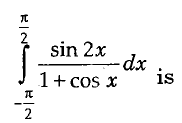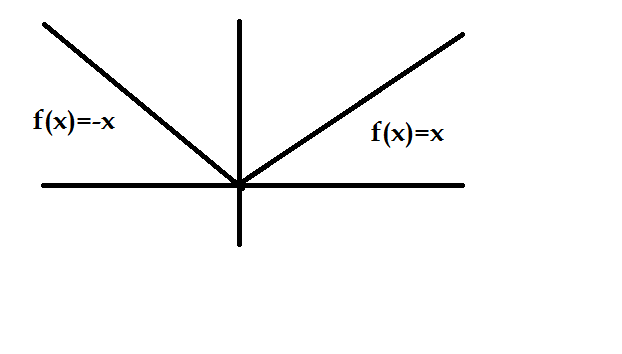# Calculus - Calculus MCQ

6:

The minimum value of | x2 _ 5x + 21 | is

 A. -5 B. 0 C. -1 D. -2 Answer Report Discuss Option: B Explanation : Since value of a mod function cannot be less than zero, therefore f(x) = | x2 - 5x + 21 | is zero. Click on Discuss to view users comments. Write your comments here:
7:

The value of the improper integral

 A. 1/4 B. 0 C. -1/4 D. 1 Answer Report Discuss Option: C Explanation : Click on Discuss to view users comments. Write your comments here:
8:

Value of the definite integralA. -2ln2 B. 2 C. 0 D. (ln2)2 Answer Report Discuss Option: D Explanation : Click on Discuss to view users comments. Write your comments here:
9:

The function f(x) = 3x(x - 2) has a

 A. minimum at x = 1 B. maximum at x = 1 C. minimum at x = 2 D. maximum at x = 2 Answer Report Discuss Option: A Explanation : f(x) = 3x (x - 2) = 3x2 - 6x Differentiating, we getAgain diferentiating,For maxima or minima, - f '(x) =0 ∴  6x-6=0  ⇒ x=1 As f '(x) =6 >0 then f(x) is minimum at x=1   Click on Discuss to view users comments. Write your comments here:
10:

What is the derivative of f(x) =  | x | at x = 0

 A. 1 B. -1 C. 0 D. Does not exist Answer Report Discuss Option: D Explanation : f(x) = |x| and f(x) = x for x > 0 ⇒f(x) = -x for x < 0Hence from the graph it is clear that derivative doesn't exists for x=0 Click on Discuss to view users comments. Write your comments here: